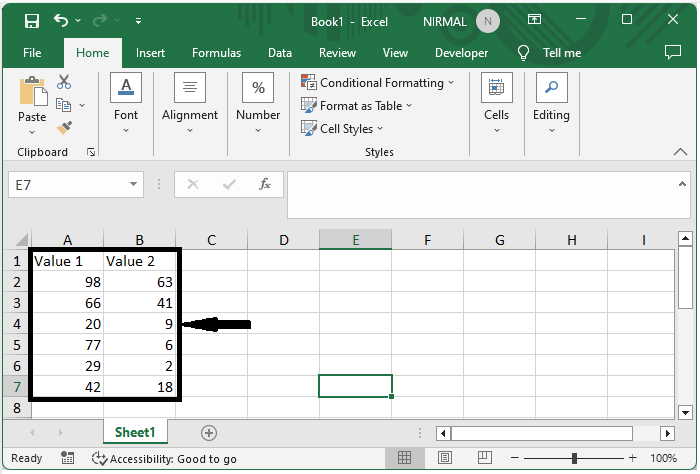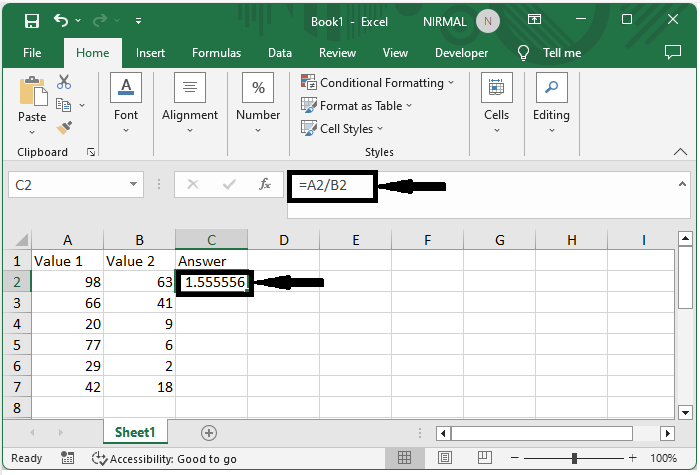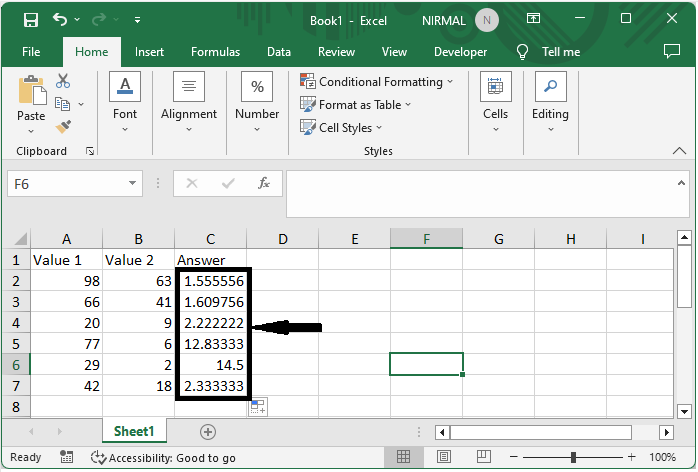# How To Divide One Column By Another Column In Excel?

Excel is a sophisticated application that lets you do a variety of calculations and data manipulations. When you need to calculate ratios, percentages, or other types of mathematical operations on your data, dividing one column by another can be extremely handy. In this article, we will look at different ways to divide one column by another in Excel. Excel contains various formulae and approaches to assist you obtain your desired outcomes, whether you have numeric numbers, percentages, or even text.

We'll go through the fundamental division formula as well as more advanced techniques like using the Paste Special tool, generating a calculated field in a pivot table, and using array formulas. Furthermore, we will go through common issues and faults that may happen during the process, as well as how to troubleshoot them. By the end of this course, you will have a firm grasp on how to divide one column by another in Excel, as well as the ability to apply this skill to your own data analysis and calculations. So let's get started and learn how to divide columns in Excel!

## Divide One Column By Another Column

Here we will first get the result, then use the autofill handle to complete the task. So let us see a simple process to know how you can divide one column by another in Excel.

### Step 1

Consider an Excel sheet where you have two lists of numbers similar to the below image.First, click on an empty cell, in our case, cell C2, and enter the formula as =B2/A2 and click enter.

Empty cell > Formula > Enter.### Step 2

Then drag down using the autofill handle to complete the task.## Conclusion

In this tutorial, we have used a simple example to demonstrate how you can divide one column by another in Excel to highlight a particular set of data.

Updated on: 12-Jul-2023

1K+ Views